##### physics ch 5

0
Set Details Share
created 2 years ago by Elena_Kei
3,260 views
Page to share:
Embed this setcancel
COPY
code changes based on your size selection
Size:
X
1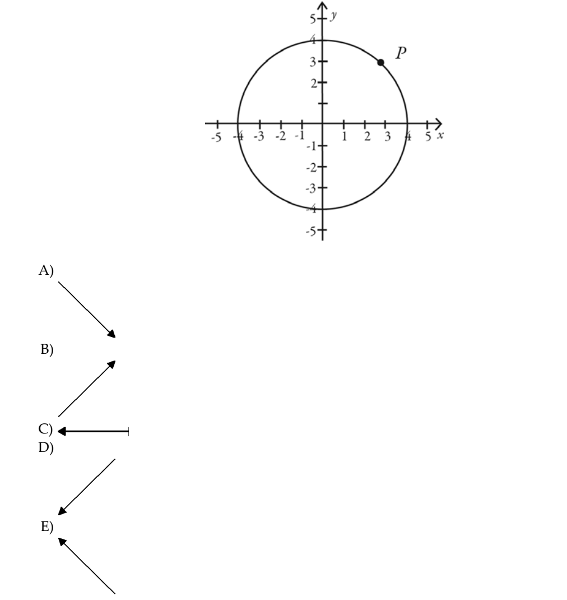1) Point P in the figure indicates the position of an object traveling at constant speed clockwise
around the circle. Which arrow best represent the direction the object would travel if the net
external force on it were suddenly reduced to zero?

D

2

An object is moving to the right, and experiencing a net force that is directed to the right. The
magnitude of the force is decreasing with time. The speed of the object is
A) increasing.
B) decreasing.
C) constant in time.

A

3

A stalled car is being pushed up a hill at constant velocity by three people. The net force on the
car is
A) zero.
B) up the hill and equal to the weight of the car.
C) down the hill and equal to the weight of the car.
D) up the hill and greater than the weight of the car.
E) down the hill and greater than the weight of the car.

A

4

4) A 615 N student standing on a scale in an elevator notices that the scale reads 645 N. From this
information, the student knows that the elevator must be moving
A) downward.
B) upward.
C) You cannot tell if it is moving upward or downward.

C

5

5) A car is being towed at constant velocity on a horizontal road using a horizontal chain. The
tension in the chain must be equal to the weight of the car in order to maintain constant
velocity.
A) True
B) False

B

6

6) You are standing in a moving bus, facing forward, and you suddenly fall forward as the bus
comes to an immediate stop. The force acting on you that causes you to fall forward is
A) the force of gravity.
B) the normal force due to your contact with the floor of the bus.
C) the force due to static friction between you and the floor of the bus.
D) the force due to kinetic friction between you and the floor of the bus.
E) No forces were acting on you to cause you to fall.

E

7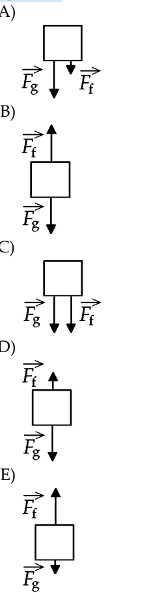7) Which one of the following free-body diagrams best represents the free-body diagram, with
correct relative force magnitudes, of a person in an elevator that is traveling upward with an
unchanging velocity? F f is the force of the floor on the person and F g is the force of gravity on
the person.

b

8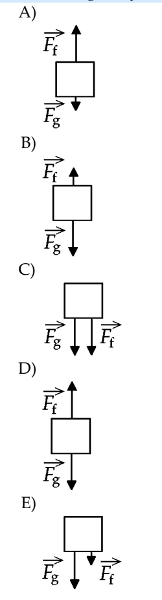8) Which one of the following free-body diagrams best represents the free-body diagram, with
correct relative force magnitudes, of a person in an elevator that is traveling upward but is
gradually slowing down at a rate of 9 m/s2? F f is the force of the floor on the person and F g is
the force of gravity on the person.

B

9

9) A crate is sliding down an inclined ramp at a constant speed of 0.55 m/s. The vector sum of all
the forces acting on this crate must point down the ramp.
A) True
B) False

B

10

10) A woman is straining to lift a large crate, without success because it is too heavy. We denote
the forces on the crate as follows: P is the upward force the woman exerts on the crate, C is the
vertical contact force exerted on the crate by the floor, and W is the weight of the crate. How
are the magnitudes of these forces related while the woman is trying unsuccessfully to lift the
crate?
A) P + C = W
B) P + C < W
C) P + C > W
D) P = C

A

11

11) Suppose the force of the air drag on an object is proportional to the speed of the object and in
the direction opposite the objectʹs velocity. If you throw an object upward, the magnitude of its
acceleration is greatest
A) right after the object is released.
B) at the top of its trajectory.
C) The acceleration of the object is the same throughout the entire trajectory.

a

12

12) An object is moving forward with a constant velocity. Which statement about this object
MUST be true?
A) The net force on the object is zero.
B) The net force on the object is in the forward direction.
C) No forces are acting on the object.
D) The acceleration of the object is in the forward direction.

a

13

13) Suppose you are playing hockey on a new‐age ice surface for which there is no friction
between the ice and the hockey puck. You wind up and hit the puck as hard as you can. After
the puck loses contact with your stick, the puck will
A) start to slow down.
B) not slow down or speed up.
C) speed up a little, and then slow down.
D) speed up a little, and then move at a constant speed.

b

14

14) A ball is tossed vertically upward. When it reaches its highest point (before falling back
downward)
A) the velocity is zero, the acceleration is directed downward, and the force of gravity acting
on the ball is directed downward.
B) the velocity is zero, the acceleration is zero, and the force of gravity acting on the ball is
zero.
C) the velocity is zero, the acceleration is zero, and the force of gravity acting on the ball is
directed downward.
D) the velocity and acceleration reverse direction, but the force of gravity on the ball
remains downward.
E) the velocity, acceleration, and the force of gravity on the ball all reverse direction.

A

15

15) A dog is standing in the bed of a pickup truck. The bed is coated with ice, causing the force of
friction between the dog and the truck to be zero. The truck is initially at rest, and then
accelerates to the right, moving along a flat road. As seen from a stationary observer (watching
the truck move to the right), the dog
A) does not move left or right, but the back of the truck moves towards the dog.
B) moves to the right, but not as quickly as the truck is moving to the right, causing it to
slide towards the back of the truck.
C) moves to the right at the same rate as the truck, so it doesnʹt slide.
D) moves to the left, as the truck moves to the right, causing the dog to slide towards the
back of the truck.

a

16

16) You are seated in a bus and notice that a hand strap that is hanging from the ceiling hangs
away from the vertical in the backward direction. From this observation, you can conclude
that
A) the velocity of the bus is forward.
B) the velocity of the bus is backward.
C) You cannot conclude anything about the direction of the velocity of the bus.

c

17

1) If a 5.0 kg box is pulled simultaneously by a 10.0 N force and a 5.0 N force, then its acceleration
must be
A) 3.0 m/s2.
B) 2.2 m/s2.
C) 1.0 m/s2.
D) We cannot tell from the information given.

d

182) The figure shows an acceleration-versus-force graph for three objects pulled by rubber bands.
The mass of object 2 is 36 kg. What are the masses of objects 1 and 3?

A) 14 kg and 90 kg
B) 72 kg and 18 kg
C) 90 kg and 18 kg
D) 14 kg and 72 kg

A

19

A 7.0-kg object is acted on by two forces. One of the forces is 10.0 N acting toward the east.
Which of the following forces is the other force if the acceleration of the object is 1.0 m/s2
toward the east?
A) 6.0 N east
B) 3.0 N west
C) 12 N east
D) 9.0 N west
E) 7.0 N west

B

20

4) An 1100-kg car traveling at 27.0 m/s starts to slow down and comes to a complete stop in 578
m. What is the magnitude of the average braking force acting on the car?
A) 690 N
B) 550 N
C) 410 N
D) 340 N

A

21

5) On its own, a certain tow-truck has a maximum acceleration of 3.0 m/s2. What would be the
maximum acceleration when this truck was towing a bus of twice its own mass?
A) 2.5 m/s2
B) 2.0 m/s2
C) 1.5 m/s2
D) 1.0 m/s2

D

22

6) A child on a sled starts from rest at the top of a 15° slope. If the trip to the bottom takes 15.2 s
how long is the slope? Assume that frictional forces may be neglected.
A) 293 m
B) 586 m
C) 1130 m
D) 147 m

A

23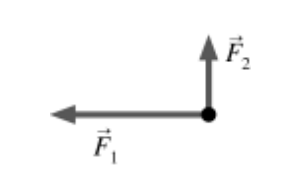7) The figure shows two forces acting at right angles on an object. They have magnitudes F1 = 6.3
N and F2 = 2.1 N. What third force will cause the object to be in equilibrium (acceleration
equals zero)?

A) 6.6 N at 162° counterclockwise from F1
B) 6.6 N at 108° counterclockwise from F1
C) 4.2 N at 162° counterclockwise from F1
D) 4.2 N at 108° counterclockwise from F 1

A

24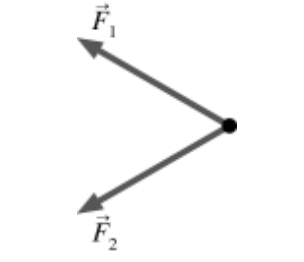8) The figure shows two forces, each of magnitude 4.6 N, acting on an object. The angle between
these forces is 40°, and they make equal angles above and below the horizontal. What third
force will cause the object to be in equilibrium (acceleration equals zero)?

A) 8.6 N pointing to the right
B) 7.0 N pointing to the right
C) 4.3 N pointing to the right
D) 3.5 N pointing to the right

A

25

An object weighing 4.00 N falls from rest subject to a frictional drag force given by Fdrag =
bv2, where v is the speed of the object and b = 3.00 N · s2/m2. What terminal speed will this
object approach?
A) 1.78 m/s
B) 3.42 m/s
C) 1.15 m/s
D) 2.25 m/s
E) 0.75 m/s

c

26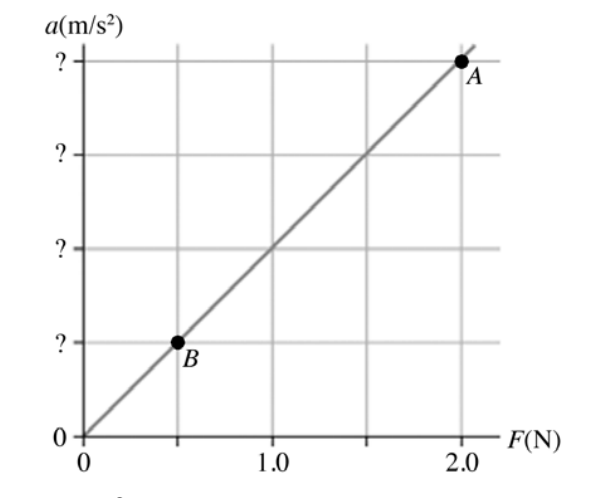The figure shows a graph of the acceleration of a 125-g object as a function of the net force
acting on it. What is the acceleration at points A and B?

A: 16 m/s^2, B: 4.0 m/s^2

27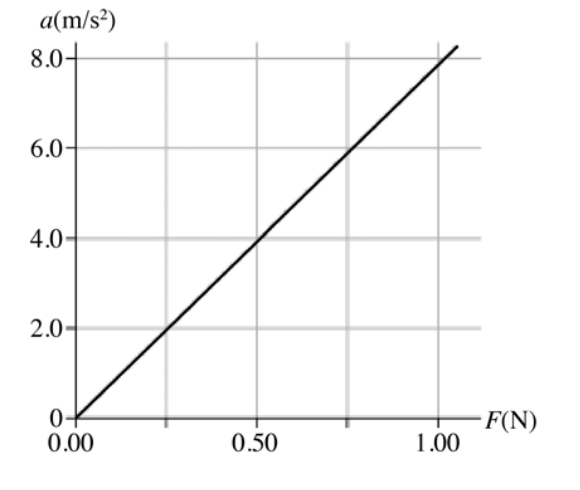The figure shows a graph of the acceleration of an object as a function of the net force acting on
it. The mass of this object, in grams, is closest to

A) 130.
B) 11.
C) 89.
D) 8000.

A

28

Two forces act on a 55-kg object. One force has magnitude 65 N directed 59° clockwise from
the positive x-axis, and the other has a magnitude 35 N at 32° clockwise from the positive
y-axis. What is the magnitude of this objectʹs acceleration?
A) 1.1 m/s2
B) 1.3 m/s2
C) 1.5 m/s2
D) 1.7 m/s2

A

29

13) The figure shows two forces acting on an object, with magnitudes F1 = 78 N and
F2 = 26 N.What third force will cause the object to be in equilibrium (acceleration equals
zero)?

A) 52 N pointing down
B) 52 N pointing up
C) 82 N pointing down
D) 82 N pointing up

A

30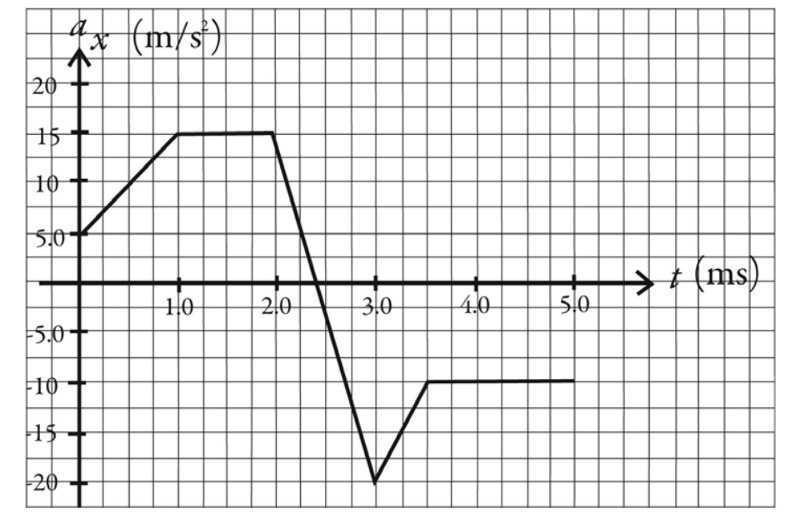The graph in the figure shows the x component of the acceleration of a 2.4-kg object as a
function of time (in ms).

(a) At what time(s) does the x component of the net force on the object reach its maximum
magnitude, and what is that maximum magnitude?
(b) What is the x component of the net force on the object at time t = 0.0 ms and at t = 4.0 ms?

Answer: (a) At 3.0 ms, 48 N (b) 12 N, -24 N

3115) The graph in the figure shows the net force acting on a 3.0-kg object as a function of time.

(a) What is the acceleration of this object at time t = 2.0 s?
(b) Draw, to scale, a graph of the acceleration of this object as a function of time over the range
t = 0.00 s to t = 7.0 s.

Answer: (a) 2.0 m/s2
(b) The acceleration-time graph looks the same as the force-time graph except on the
vertical axis the numbers (starting at 2.0) are replaced by 0.67, 1.3, 2.0, 2.7, 3.3, and 4.0.